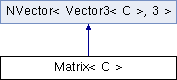ParM  parm A molecular dynamics library
Matrix< C > Class Template Reference

A 3x3 matrix, with methods for adding, subtracting, dot product, etc. More...

#include <vec.hpp>

Inheritance diagram for Matrix< C >:## Public Member Functions

Vector3< C > dot (Vector3< C > v) const

Vector3< C > operator* (Vector3< C > v) const

Matrix< C > SymmetricInverse () const
The inverse of a symmetric inverse. More...

det () const
The determinant. More...Public Member Functions inherited from NVector< Vector3< C >, 3 >
NVector ()

NVector (const NVector &rhs)

NVector (const NVector< U, N > &rhs)

NVector (const Vector3< C > locs[N])

const Vector3< C > & get (const unsigned int n) const

void set (const unsigned int n, const Vector3< C > a)

unsigned int len () const

NVectoroperator+= (const NVector &rhs)

NVectoroperator-= (const NVector &rhs)

NVectoroperator*= (const U rhs)

NVectoroperator/= (const U rhs)

NVector operator- () const

NVector operator- (const NVector &rhs) const

NVector operator+ (const NVector &rhs) const

Vector3< C > & operator[] (const unsigned int i)

const Vector3< C > & operator[] (const unsigned int i) const

NVector operator* (const U rhs) const
Multiplication by a scalar. More...

NVector operator/ (const U rhs) const
Division by a scalar. More...

Vector3< C > * begin ()

Vector3< C > * end ()

~NVector ()

## Additional Inherited MembersPublic Types inherited from NVector< Vector3< C >, 3 >
typedef Vector3< C > * iteratorProtected Attributes inherited from NVector< Vector3< C >, 3 >
Vector3< C > vals [N]

## Detailed Description

### template<class C> class Matrix< C >

A 3x3 matrix, with methods for adding, subtracting, dot product, etc.

Useful for rotations.

Template Parameters
 T a numerical type, as as float or double.

## Member Function Documentation

template<class C >
 C Matrix< C >::det ( ) const

The determinant.

template<class C >
 Vector3< C > Matrix< C >::dot ( Vector3< C > v ) const
template<class C>
 Vector3 Matrix< C >::operator* ( Vector3< C > v ) const
inline
template<class C >
 Matrix< C > Matrix< C >::SymmetricInverse ( ) const

The inverse of a symmetric inverse.

The documentation for this class was generated from the following file: